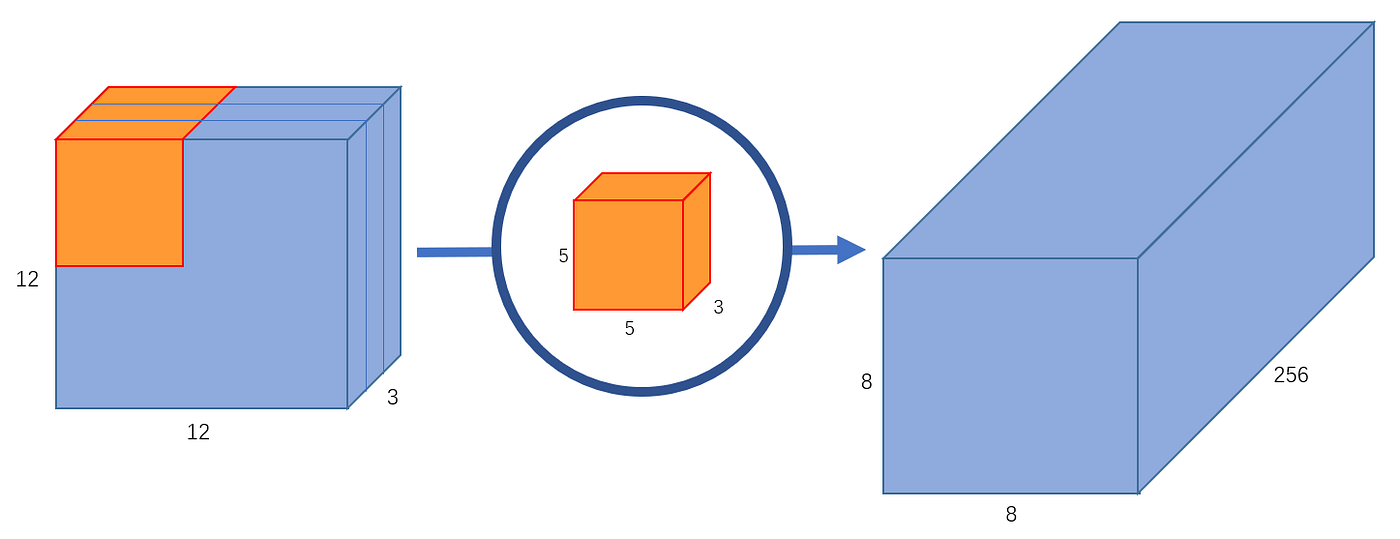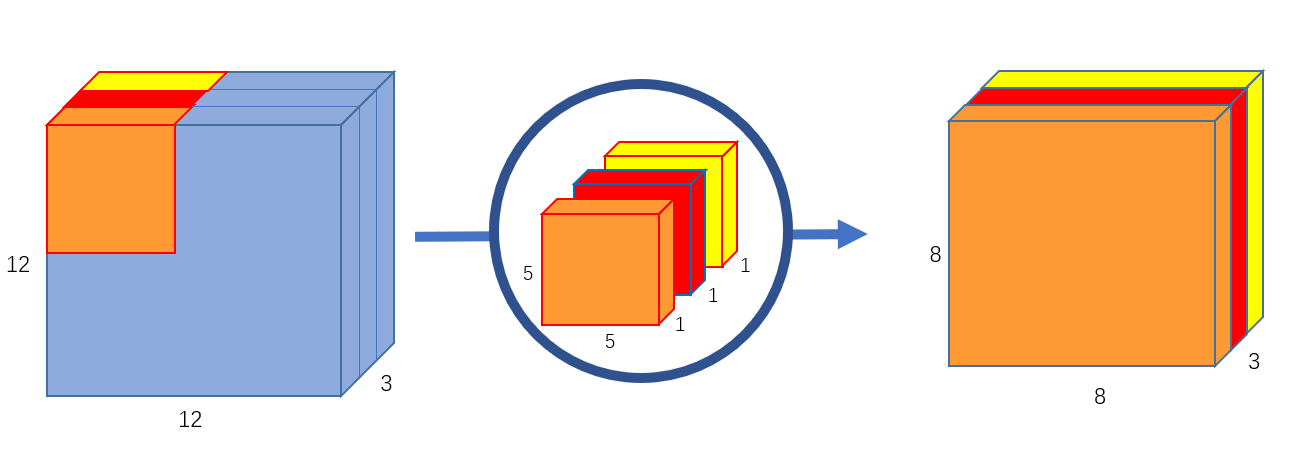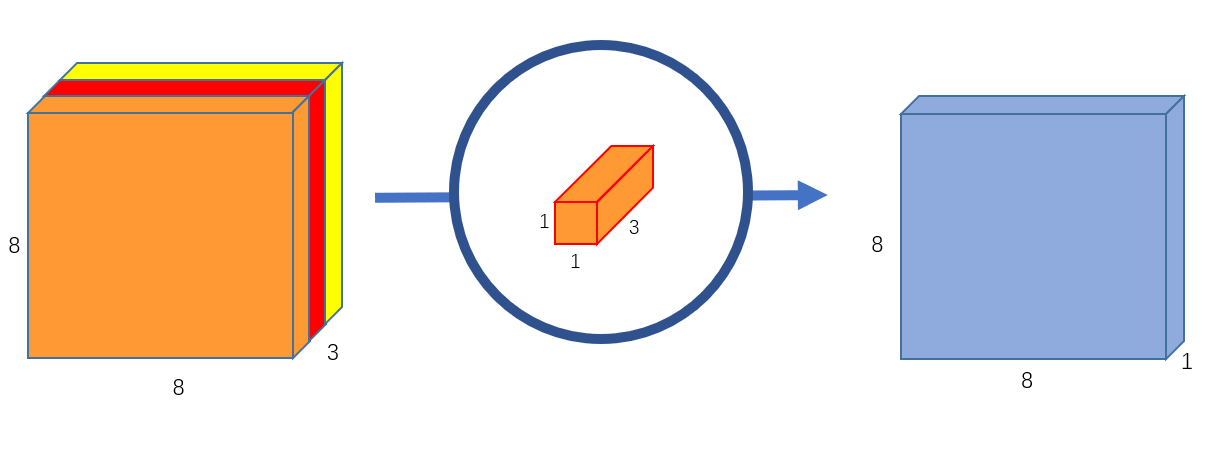# Efficient Convolutional Neural Network with MobileNet

## Problem

The number of operations of convolution is quite resource-intensive. Suppose we have an input image of size $$D_x \times D_x \times M$$ and a convolution block with a kernel of size $$D_k \times D_k \times M$$. First, the conv block will do element-wise multiplication with a segment on the input image. Then, we sum the value along all the channels into a single value. The kernel then slides by one block to the right (if the stride is 1), and the whole process is repeated until all ceils are covered. $$N$$ kernel is used to obtain $$N$$ channel as output.Regular Convolution (Source: https://towardsdatascience.com/a-basic-introduction-to-separable-convolutions-b99ec3102728)

Operations of a regular Conv block are:

• One Conv Block's Operations: $$D_k \cdot D_k \cdot M$$

• One Block's Slide over the input: $$D_x \cdot D_x$$

Using $$N$$ conv blocks, the total operation is:

$$T = D_k \cdot D_k \cdot M \cdot D_x \cdot D_x \cdot N$$

## Solution

One key challenge is that the conv multiplication is repeated for all $$N$$ blocks. For example, one conv block is applied to the top-left corner of the input. The second block will re-compute everything again. The idea of a Depth-wise separable convolution block is to not repeat that element-wise multiplication.

Each of the regular conv blocks does multiplication followed by additions. In depth-wise separable convolution, the process is split into two. One layer is for multiplication and another is for addition.

• Depthwise Convolution: This is similar to regular conv block except it does not perform additional across all channels. Hence, the operation is $$D_k \cdot D_k \cdot M \cdot D_x \cdot D_x$$.Depthwise Convolution (Source: https://towardsdatascience.com/a-basic-introduction-to-separable-convolutions-b99ec3102728)

• Pointwise Convolution: This is a $$1 \times 1 \times M$$ kernel. $$N$$ kernels are used to obtain $$N$$ channels as output. The number of operations: $$D_x \cdot D_x \cdot M \cdot N$$.Pointwise Convolution (Source: https://towardsdatascience.com/a-basic-introduction-to-separable-convolutions-b99ec3102728)

Total operations of Depth-wise Separable Convolution:

$$(D_k \cdot D_k \cdot M \cdot D_x \cdot D_x) + (D_x \cdot D_x \cdot M \cdot N)$$

Let's compare:

$$\frac{(D_k \cdot D_k \cdot M \cdot D_x \cdot D_x) + (D_x \cdot D_x \cdot M \cdot N)}{D_k \cdot D_k \cdot M \cdot D_x \cdot D_x \cdot N} =\frac{1}{N} + \frac{1}{D_k \cdot D_k}$$

Here we can see that the depth-wise approach requires way fewer operations than the regular conv block.

## How to use

Instead of using a regular convolution layer, we use two separate operations - depthwise and pointwise convolutions.Regular Conv vs. Depthwise Separable Conv (Source: Original research paper)

## References

MobileNets: Efficient Convolutional Neural Networks for Mobile Vision Applications(Howard. et al, 2017)